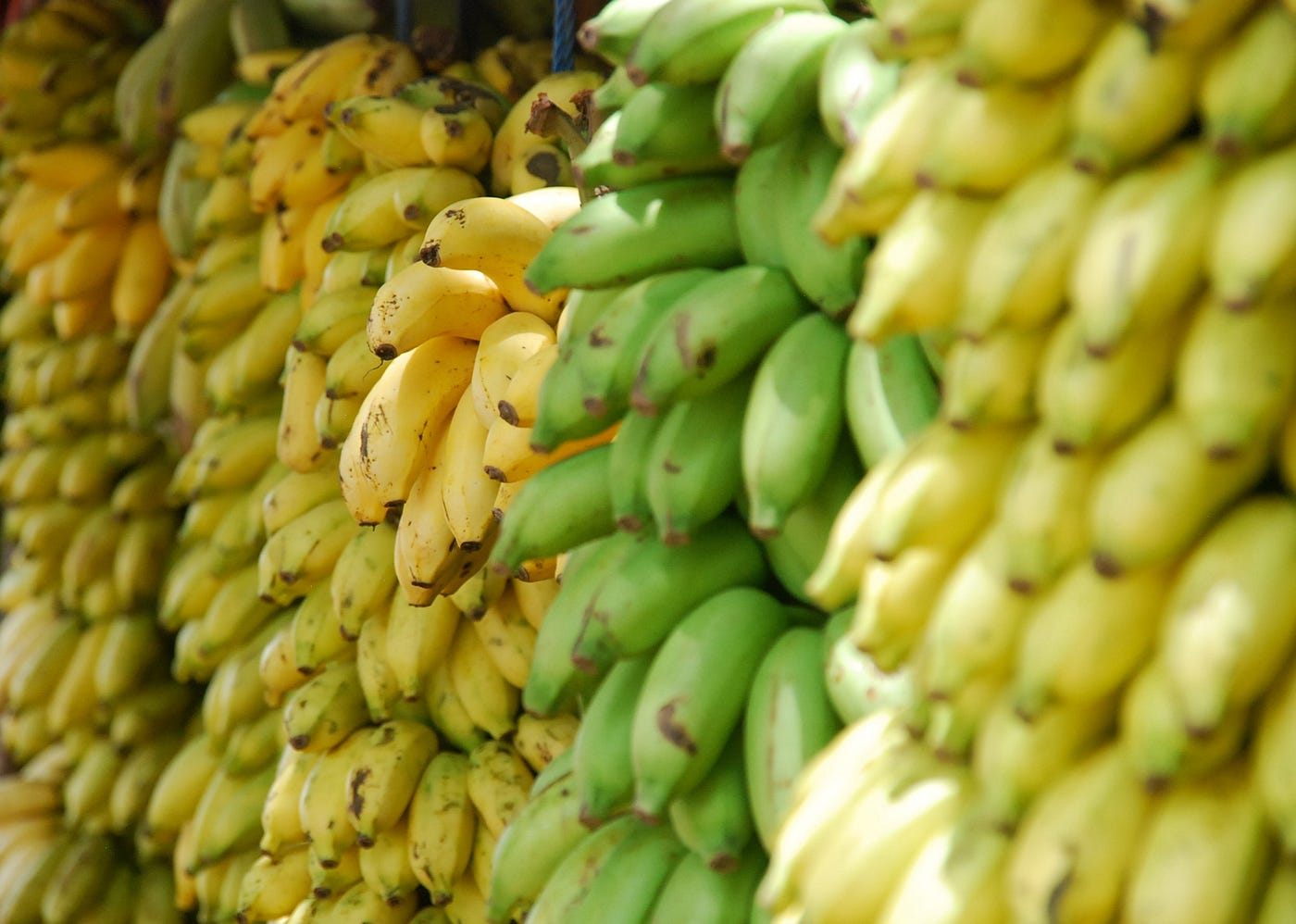# Koko Eating BananasPhoto by Lotte Löhr on Unsplash

Koko loves to eat bananas. There are `n` piles of bananas, the `ith` pile has `piles[i]` bananas. The guards have gone and will come back in `h` hours.

Koko can decide her bananas-per-hour eating speed of `k`. Each hour, she chooses some pile of bananas and eats `k` bananas from that pile. If the pile has less than `k` bananas, she eats all of them instead and will not eat any more bananas during this hour.

Koko likes to eat slowly but still wants to finish eating all the bananas before the guards return.

Return the minimum integer `k` such that she can eat all the bananas within `h` hours.

Example 1:

`Input: piles = [3,6,7,11], h = 8Output: 4`

Example 2:

`Input: piles = [30,11,23,4,20], h = 5Output: 30`

Example 3:

`Input: piles = [30,11,23,4,20], h = 6Output: 23`

Constraints:

• `1 <= piles.length <= 104`
• `piles.length <= h <= 109`
• `1 <= piles[i] <= 109`

Algorithm-

We can use Binary Search to find the minimum `k` -

• if `hours > h`, that indicates `k` is too small, then `low = k + 1`
• if `hours < h`, that indicates `k` is too large, then `high = k - 1`
• if `hours == h`, we can try a smaller `k`, then also `high = k - 1`
• intuitively, we can initialize `low = 1, high = 1000000000` or `low = 1, high = max(piles)`
• Note that we are searching `k` via Binary Search, we need not sort any array or list, the condition of Binary Search is
• The search space is limited
• Every time after checking for the current `mid`, we know exactly where to search next (greater than `mid` or lower than `mid`)
• Space complexity: O(1)
• Time complexity: O(n*log(max(piles)))

--

--